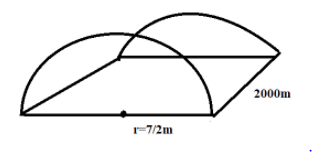The length of a semi-circular tunnel is 2 km and diameter is 7 m. Find the expenditure for digging the tunnel at the rate of Rs. 600 per m 3  . Find the expenditure for plastering inner side of the tunnel at the rate of Rs. 50 per  m 2  . π= 22 7

# The length of a semi-circular tunnel is 2 km and diameter is 7 m. Find the expenditure for digging the tunnel at the rate of Rs. 600 per  . Find the expenditure for plastering inner side of the tunnel at the rate of Rs. 50 per   .

1. A2. B3. C4. D
Rs, 2.10.000

Fill Out the Form for Expert Academic Guidance!l

+91

Live ClassesBooksTest SeriesSelf Learning

Verify OTP Code (required)

### Solution:

Given, length of the semi-circular tunnel, say    is equal to 2km, which is equal to 2000m.
And the diameter of the tunnel is 7m.
We know that the diameter is double the radius.
Then, the radius of the semi-circular tunnel, say   is  .Now, the volume of the semi-circle is half the volume of the circular tunnel.
Therefore, the volume of the circular tunnel is
Substituting the values,

Then, the expenditure of digging the tunnel at the rate of Rs. 600 per  .

So, option 4 is correct.

## Related content

 Area of Square Area of Isosceles Triangle Pythagoras Theorem Triangle Formula Perimeter of Triangle Formula Area Formulae Volume of Cone Formula Matrices and Determinants_mathematics Critical Points Solved Examples Type of relations_mathematics+91

Live ClassesBooksTest SeriesSelf Learning

Verify OTP Code (required)